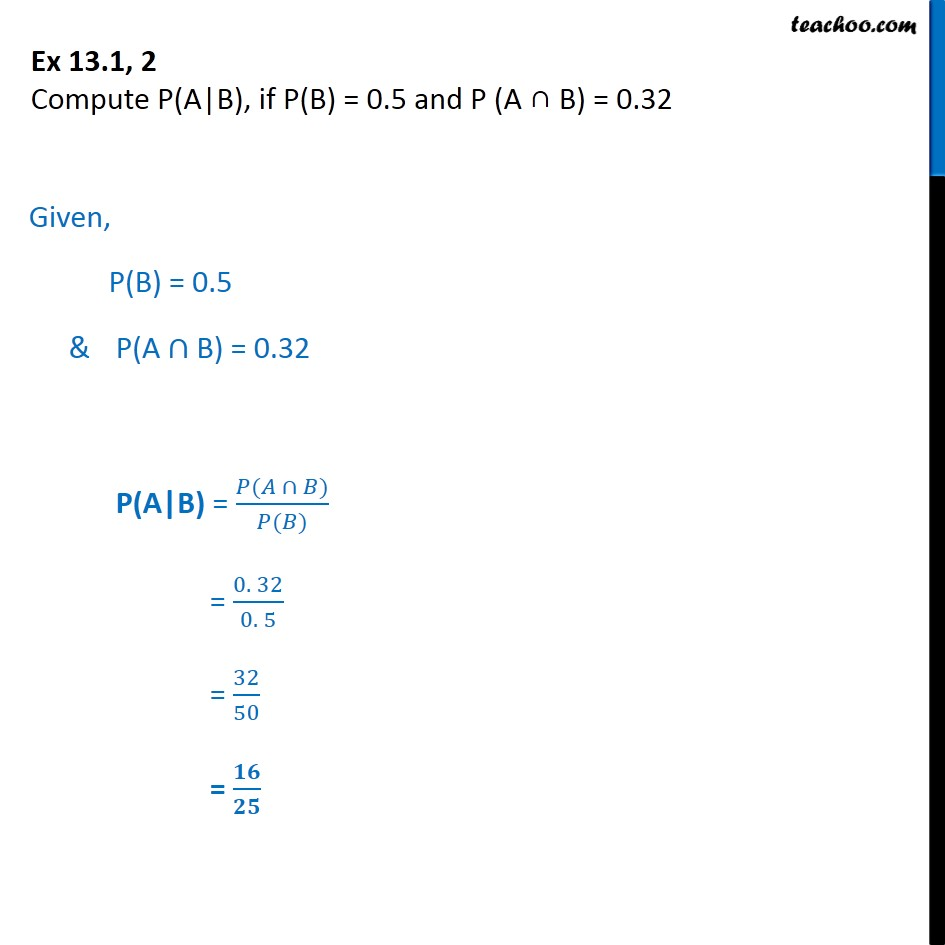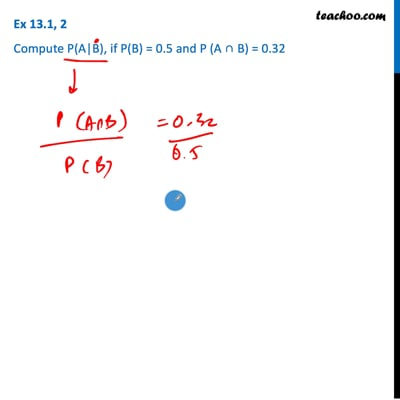Ex 13.1

Chapter 13 Class 12 Probability
Serial order wiseThis video is only available for Teachoo black users

Solve all your doubts with Teachoo Black (new monthly pack available now!)

### Transcript

Ex 13.1, 2 Compute P(A|B), if P(B) = 0.5 and P (A B) = 0.32 Given, P(B) = 0.5 & P(A B) = 0.32 P(A|B) = ( ) ( ) = 0. 32 0. 5 = 32 50 =